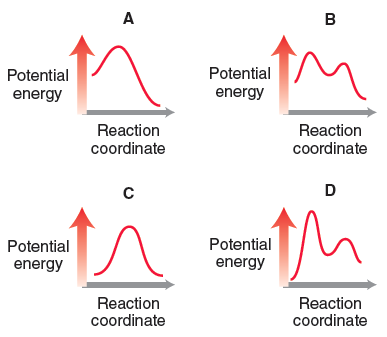# Problem: Consider the following four energy diagrams:Which processes will have a value of K eq that is greater than 1?

###### FREE Expert Solution
97% (426 ratings)
###### Problem Details

Consider the following four energy diagrams:

Which processes will have a value of K eq that is greater than 1?Frequently Asked Questions

What scientific concept do you need to know in order to solve this problem?

Our tutors have indicated that to solve this problem you will need to apply the Chemical Equilibrium concept. If you need more Chemical Equilibrium practice, you can also practice Chemical Equilibrium practice problems.

What is the difficulty of this problem?

Our tutors rated the difficulty ofConsider the following four energy diagrams:Which processes ...as high difficulty.

What professor is this problem relevant for?

Based on our data, we think this problem is relevant for Professor Pillai's class at ASU.

What textbook is this problem found in?

Our data indicates that this problem or a close variation was asked in Organic Chemistry - Klein 1st Edition. You can also practice Organic Chemistry - Klein 1st Edition practice problems.